Cursed Treasure (Answer) by Jay Lorch and Michelle Teague

There are many ways to figure out a rule, but here's the one we like best. Lay out the treasures that have that curse on your left side; lay out the others on your right side. Look for what one side has that the other side doesn't have. Also, look for a pair of treasures, one on each side, that are nearly the same—their differences may suggest what the rule is.

You should find the rules are as follows: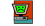A treasure has this curse if and only if it has no red gems.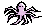A treasure has this curse if and only if it has no two touching gems.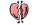A treasure has this curse if and only if it has gems of three or more colors.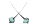A treasure has this curse if and only if it has vertical symmetry.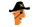A treasure has this curse if and only if it has more large gems than small gems.

Each curse's description contains a bold part that suggests a number, as follows.Computer hex refers to the hexadecimal system, whose base is 16.An octopus has 8 legs.Quadruple means multiplied by 4.Dual means pertaining to 2 things.One is obviously 1.

For each unlabelled treasure, you can apply the rules above to figure out which curses it has. Then, by adding the numbers for all those curses, you can get a total for each treasure, as follows: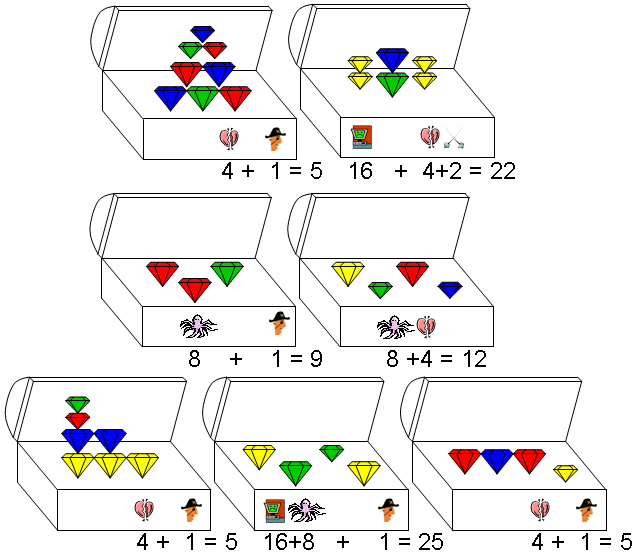The resulting totals are 5, 22, 9, 12, 5, 25, 5. Converting each into a letter using the standard approach of indexing into the alphabet gives E, V, I, L, E, Y, E. Thus, the answer is EVIL EYE.